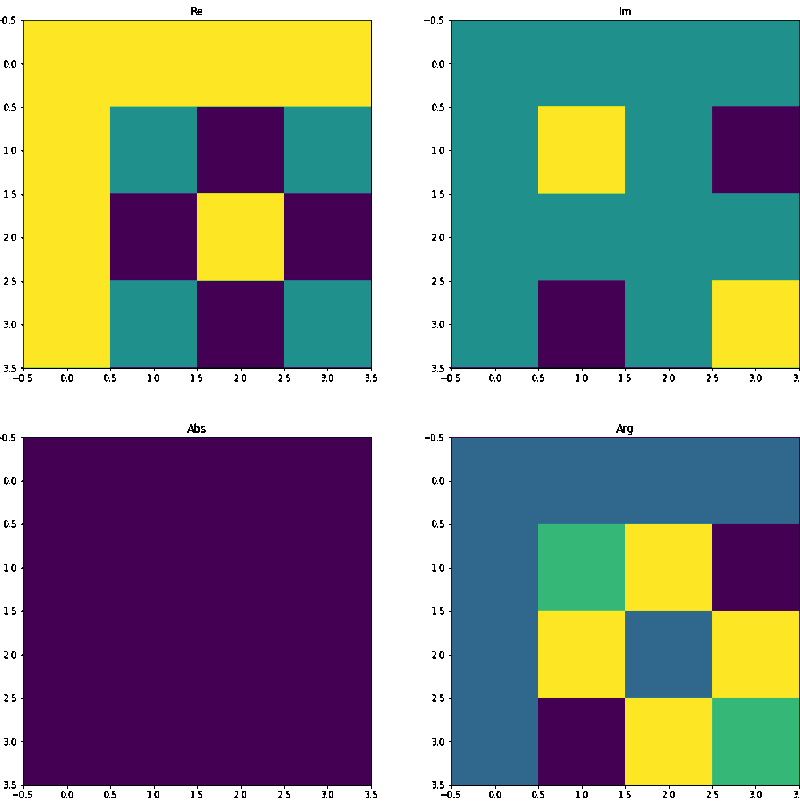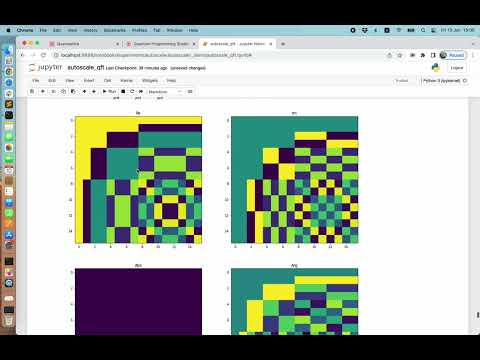Quantum Algorithm Generator

# Automatic Design of Quantum Algorithms

### The development of quantum algorithms is a job for machines — not for humans

In order to solve hard computational problems, only having a fault-tolerant quantum computer is not enough. You’ll need an algorithm as well, and that is not trivial.

# The problem

Quantum computers are very different from classical digital computers, so the approach to an algorithm is very different too. In order to design a quantum algorithm, it is necessary to tame quantum mechanical phenomena such as superposition, entanglement and interference. The trouble is, quantum mechanics challenges our intuition, so scientists struggle to figure out the best algorithms for performing meaningful tasks.

## Designing a new quantum algorithm is hard

Today, after years of research, we still have only a few quantum algorithms which promise speed-up over their classical counterparts. Quantum computers are improving and the number of qubits is growing every year. But with more qubits the problem with algorithms is getting bigger. To achieve quantum advantage we need to design quantum circuits with hundreds, and soon with thousands, maybe millions of qubits. And this is… hard!

## Automated approach

But, tasks that are hard for humans could be easy for robots, so we at Quantastica decided to try to automate the quantum algorithm design process. And after 2 years of development the Quantum Algorithm Generator was born, and the results are more than promising.

# First results

Our beta testers and we have already recorded interesting results. To mention a few:

## Scaling to 100’s of Qubits

June 2022

We have demonstrated the ability to automatically design and scale-up simple quantum algorithm primitives to many qubits. See the video here: https://youtu.be/qk7biBpNHcY.

## Scientific paper by ORNL

February 2021

Researchers from Oak Ridge National Laboratory (ORNL) used our tool to develop quantum circuits for elementary particle simulation: arXiv:2008.08763v3 [quant-ph] 17 Feb 2021 (see ref. ).

## Automatically solved IBM Q Challenge

May 2020

IBM Quantum Challenge was graded for circuit cost. Our tool found the perfect solution in only a few seconds. See the video here: https://youtu.be/UdRgUO7uIbg.

# How it works?

We have automated process of quantum algorithm design. See how it works in 5 easy steps:

## Step 1: Prepare input data

Similar to classical machine learning, input to our tool is a training set. So we don’t even need to know the classical solution for the problem - all we need is example data. If we know classical function - even better, then we can generate a high quality training set and use it as input.Input into quantum algorithm generator is classical training set

## Step 2: Encode data into state vectors

From each row in a dataset (each example) we need to produce a pair of state vectors:

• in first state vector we encode input values (features)

• in second state vector we encode output values (labels)

That way, we create as many pairs of state vectors as many rows in the dataset.

At this point we need to choose how we will encode values into state vectors. This is actually the hardest part of the whole process. If you choose the wrong encoding scheme, generator will not be able to find a solution.Examples are encoded into pairs of state vectors

## Step 3: Find unitary transformation (or even better find a circuit)

At this point, we are searching for a answer to one of two questions:

1.) Which single unitary matrix multiplied with prepared state Ψ will result in final state Ψ′ for each pair?

OR

2.) Which single circuit when executed on prepared state Ψ will produce final state Ψ′ for each pair?

That is two strategies and which one we will choose depends on what we will do with the resulting circuit. If we wish to scale-up to many qubits, then we need to produce as efficient circuits as possible, so we choose strategy 2.

For each strategy, we have developed multiple solvers using different techniques:

1.) For finding unitary matrices we made an efficient algorithm, but this way we don’t directly produce a circuit: we need to decompose the resulting matrix first. Decomposition techniques usually return a sub-optimal circuit with many gates. This is not necessarily bad, but for automatic scaling in later steps, it is essential to start with optimal circuits.

2.) Instead matrix, we prefer to directly find optimal (or as efficient as possible) circuits, so we made following solvers:

• Brute force: returns optimal circuit but is limited due to the number of variations. Perfect if the problem can be solved with a small and shallow circuit.

• Heuristics: in some cases returns sub-optimal circuits, but still better than output from matrix decomposition.

• Heuristics + ML: can reach bigger circuits, but sub-optimal.Multiple solvers for reverse-engineering circuits from pairs of state vectors

## Step 4: Prepare for scaling

If we are happy with the resulting circuit then this is the end. But if we want to scale-up to many qubits, then we need to repeat steps 1–3 at least twice, each time with one qubit more. For example: if we encoded data into 4-qubit vectors then we have 4-qubit circuit(s). Now, we need to repeat the same with 5-qubits and 6-qubits.Preparing circuits with n, n+1 and n+2 qubits

## Step 5: Scale-up

Finally, when we have 3 sets of circuits (with n, n+1 and n+2 qubits), we are ready for the next step: a post processing tool which we call “Autoscaler”.

Autoscaler is exploiting two facts:

1.) If we observe unitary matrices of the same algorithm with different numbers of qubits, we can see that values are following a pattern. From the pattern it is impossible to predict exactly the next bigger matrix, but we have a good hint about it. This is useful input to our heuristics algorithm.The animation shows visualised unitary matrix of the QFT circuit from 2–8 qubits

2.) If we observe circuits with different numbers of qubits, we can spot the pattern on how gates are arranged, and thus we can predict how the next bigger circuit could be assembled. This is true only when we have optimal circuits at input (returned by brute force or heuristic solver).Automatic extrapolation

If an autoscaler can find the pattern, then it can produce a circuit with many qubits (100’s of qubits on a laptop).

# Example

In the following 5-minute video you can see the entire process: Quantum Fourier Transform (QFT) algorithm is reverse-engineered from classical Fast Fourier Transform (FFT) function. Generated dataset is encoded into 2, 3, and 4-Qubit vectors and resulting circuits are automatically scaled-up to 50 Qubits:50-qubit QFT automatically generated from classical FFT

# Current state

The tool is under active development, and our main focus is on:

• Improving vectors-to-matrix and vectors-to-circuit solvers

• Improving autoscaler

• More automation: In ideal case, users will provide dataset, and the tool will return (large) quantum circuit without human intervention (without thinking about correct encoding scheme or best solver for the case, etc.)

# Who is using the generator?

Quantum Algorithm Generator is useful tool for companies who are preparing or experimenting with quantum computing. It is also a “must have” tool for researchers and students. It saves a lot of time, and also enables people without deep knowledge of linear algebra and quantum physics to make quantum programs.

Generator is in the beta (test) stage and we offer “early bird” registrations. If you wish to become an early adopter, please sign up and we will contact you soon with further instructions.

• ### Finland office

Lapinlahdenkatu 16 C, 00180 Helsinki, Finland, EU

• ### General Enquiries

info@quantastica.com

### Customer Support

support@quantastica.com

### Partnership

partners@quantastica.com